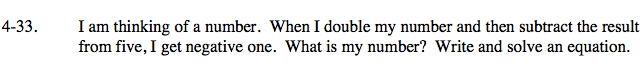### Home > CAAC > Chapter 4 > Lesson 4.1.4 > Problem4-33

4-33.Assign the number a variable.

Ex. The number = x

Put the words into equation format.

When I double my number

Then subtract the result from five

I get negative one

Solve for x.

2x

5 − 2x

5 − 2x = −1

x = 3